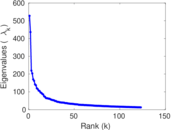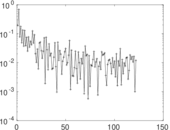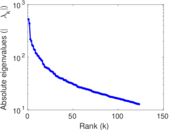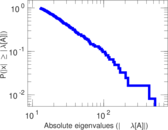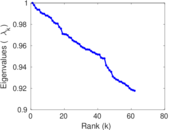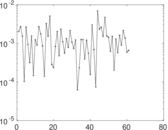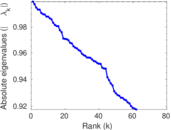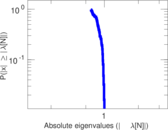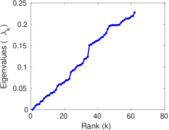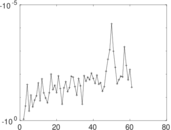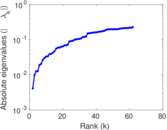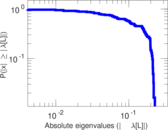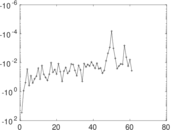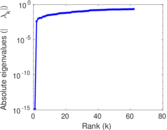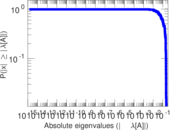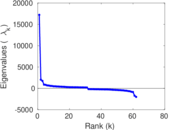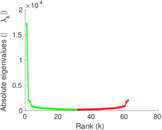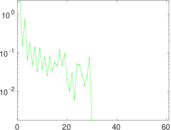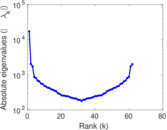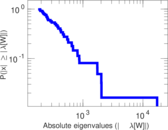# Wikipedia edits (bo)

This is the bipartite edit network of the Tibetan Wikipedia. It contains users and pages from the Tibetan Wikipedia, connected by edit events. Each edge represents an edit. The dataset includes the timestamp of each edit.

 Code `bo` Internal name `edit-bowiki` Name Wikipedia edits (bo) Data source http://dumps.wikimedia.org/ AvailabilityDataset is available for download Consistency checkDataset passed all tests Category Authorship network Dataset timestamp 2017-10-20 Node meaning User, article Edge meaning Edit Network formatBipartite, undirected Edge typeUnweighted, multiple edges Temporal dataEdges are annotated with timestamps

## Statistics

 Size n = 17,244 Left size n1 = 1,539 Right size n2 = 15,705 Volume m = 116,737 Unique edge count m̿ = 59,253 Wedge count s = 45,008,520 Claw count z = 46,750,701,602 Cross count x = 47,499,977,583,491 Square count q = 101,751,501 4-Tour count T4 = 994,179,410 Maximum degree dmax = 9,515 Maximum left degree d1max = 9,515 Maximum right degree d2max = 503 Average degree d = 13.539 4 Average left degree d1 = 75.852 5 Average right degree d2 = 7.433 11 Fill p = 0.002 451 51 Average edge multiplicity m̃ = 1.970 14 Size of LCC N = 15,020 Diameter δ = 13 50-Percentile effective diameter δ0.5 = 3.649 75 90-Percentile effective diameter δ0.9 = 5.838 13 Median distance δM = 4 Mean distance δm = 4.430 66 Gini coefficient G = 0.857 363 Balanced inequality ratio P = 0.140 358 Left balanced inequality ratio P1 = 0.063 578 8 Right balanced inequality ratio P2 = 0.187 216 Relative edge distribution entropy Her = 0.758 221 Power law exponent γ = 2.460 85 Tail power law exponent γt = 2.521 00 Tail power law exponent with p γ3 = 2.521 00 p-value p = 0.000 00 Left tail power law exponent with p γ3,1 = 1.711 00 Left p-value p1 = 0.000 00 Right tail power law exponent with p γ3,2 = 3.531 00 Right p-value p2 = 0.000 00 Degree assortativity ρ = −0.146 864 Degree assortativity p-value pρ = 6.293 11 × 10−283 Spectral norm α = 527.854 Algebraic connectivity a = 0.004 095 54 Spectral separation |λ1[A] / λ2[A]| = 1.210 21 Controllability C = 13,239 Relative controllability Cr = 0.821 737

## Plots

### Fruchterman–Reingold graph drawing### Degree distribution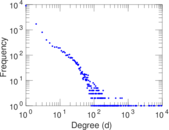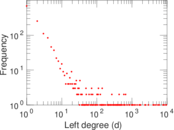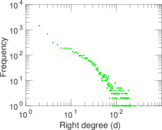### Cumulative degree distribution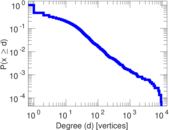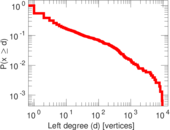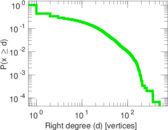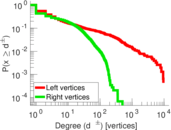### Lorenz curve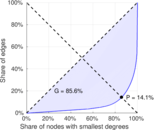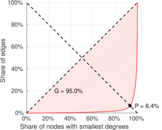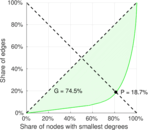### Spectral distribution of the adjacency matrix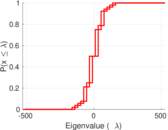### Spectral distribution of the normalized adjacency matrix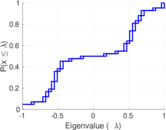### Spectral distribution of the Laplacian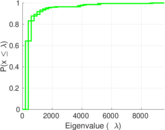### Spectral graph drawing based on the adjacency matrix### Spectral graph drawing based on the Laplacian### Spectral graph drawing based on the normalized adjacency matrix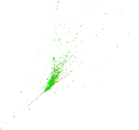### Degree assortativity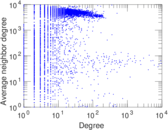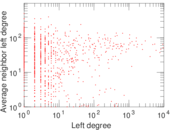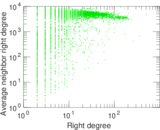### Zipf plot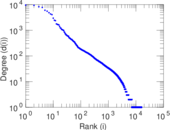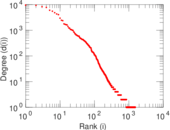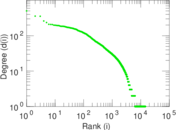### Hop distribution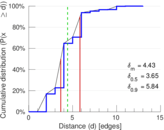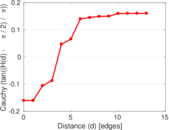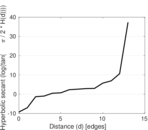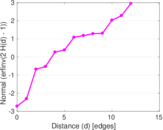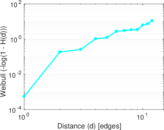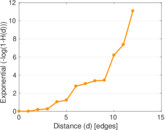### Double Laplacian graph drawing### Delaunay graph drawing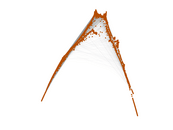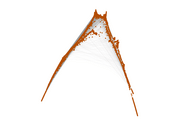### Edge weight/multiplicity distribution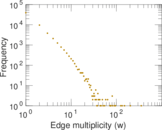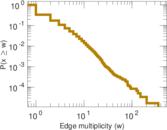### Temporal distribution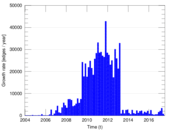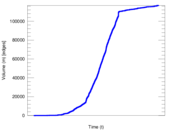### Temporal hop distribution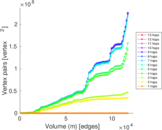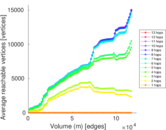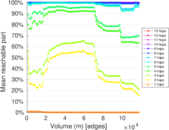### Diameter/density evolution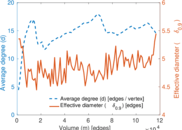### Matrix decompositions plots